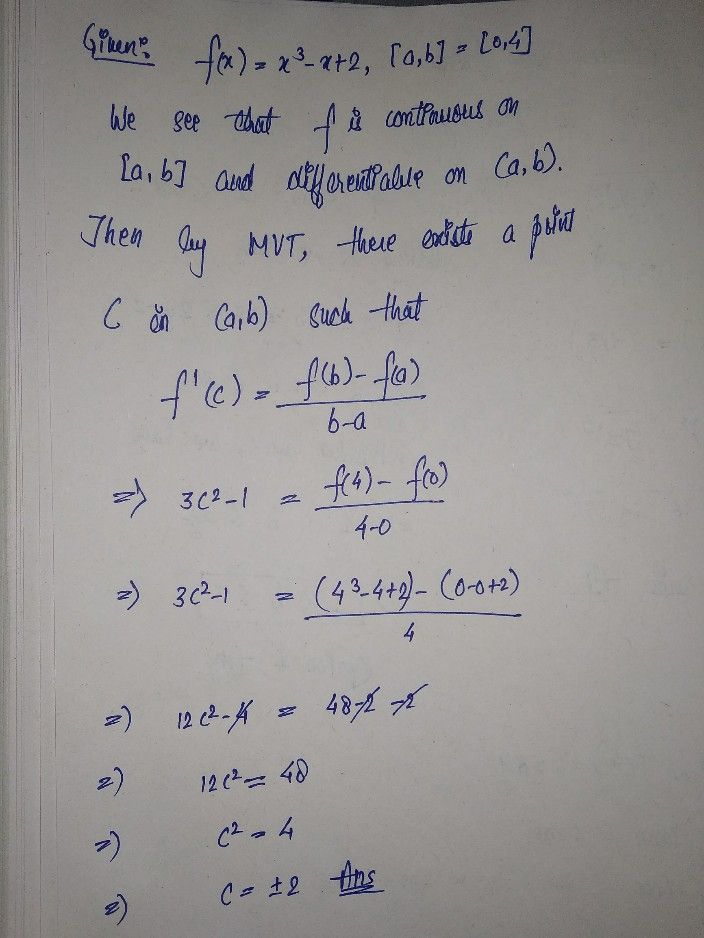Symbol
Problem$\left(4$ $4arks\right)$ (b) Find the number c which satisfies the conclusion of the Mean Value Theorem for the function $f\left(x\right)=x^{3}-x+2$ on the closed interval $\left(0,4\right)$
Calculus
SolutionQanda teacher - khubchandr